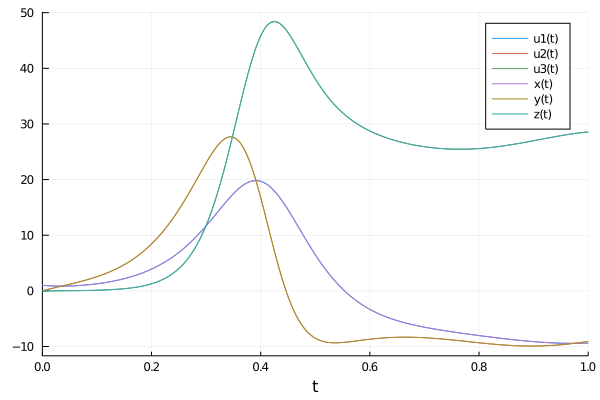# Optimising Parameters of a Lorenz System

Consider a Lorenz System ,

\begin{align*} \frac{\mathrm{d} x}{\mathrm{d}t} &= \sigma (y -x) \, ,\\ \frac{\mathrm{d} y}{\mathrm{d}t} &= x (\rho - z) - y \, ,\\ \frac{\mathrm{d} z}{\mathrm{d}t} &= x y - \beta z \, ,\\ \end{align*}

with Physics-Informed Neural Networks. Now we would consider the case where we want to optimise the parameters \sigma, \beta, and \rho.

We start by defining the the problem,

using NeuralPDE, Flux, ModelingToolkit, Optimization, GalacticOptimJL, DiffEqFlux, OrdinaryDiffEq, Plots
import ModelingToolkit: Interval, infimum, supremum
@parameters t ,σ_ ,β, ρ
@variables x(..), y(..), z(..)
Dt = Differential(t)
eqs = [Dt(x(t)) ~ σ_*(y(t) - x(t)),
Dt(y(t)) ~ x(t)*(ρ - z(t)) - y(t),
Dt(z(t)) ~ x(t)*y(t) - β*z(t)]

bcs = [x(0) ~ 1.0, y(0) ~ 0.0, z(0) ~ 0.0]
domains = [t ∈ Interval(0.0,1.0)]
dt = 0.01

And the neural networks as,

input_ = length(domains)
n = 8
chain1 = FastChain(FastDense(input_,n,Flux.σ),FastDense(n,n,Flux.σ),FastDense(n,n,Flux.σ),FastDense(n,1))
chain2 = FastChain(FastDense(input_,n,Flux.σ),FastDense(n,n,Flux.σ),FastDense(n,n,Flux.σ),FastDense(n,1))
chain3 = FastChain(FastDense(input_,n,Flux.σ),FastDense(n,n,Flux.σ),FastDense(n,n,Flux.σ),FastDense(n,1))

We will add an additional loss term based on the data that we have in order to optimise the parameters.

Here we simply calculate the solution of the lorenz system with OrdinaryDiffEq.jl based on the adaptivity of the ODE solver. This is used to introduce non-uniformity to the time series.

function lorenz!(du,u,p,t)
du = 10.0*(u-u)
du = u*(28.0-u) - u
du = u*u - (8/3)*u
end

u0 = [1.0;0.0;0.0]
tspan = (0.0,1.0)
prob = ODEProblem(lorenz!,u0,tspan)
sol = solve(prob, Tsit5(), dt=0.1)
ts = [infimum(d.domain):dt:supremum(d.domain) for d in domains]
function getData(sol)
data = []
us = hcat(sol(ts).u...)
ts_ = hcat(sol(ts).t...)
return [us,ts_]
end
data = getData(sol)
initθs = DiffEqFlux.initial_params.([chain1,chain2,chain3])
acum =  [0;accumulate(+, length.(initθs))]
sep = [acum[i]+1 : acum[i+1] for i in 1:length(acum)-1]
(u_ , t_) = data
len = length(data)

Then we define the additional loss funciton additional_loss(phi, θ , p), the function has three arguments, phi the trial solution, θ the parameters of neural networks, and the hyperparameters p .

function additional_loss(phi, θ , p)
return sum(sum(abs2, phi[i](t_ , θ[sep[i]]) .- u_[[i], :])/len for i in 1:1:3)
end

Then finally defining and optimising using the PhysicsInformedNN interface.

discretization = NeuralPDE.PhysicsInformedNN([chain1 , chain2, chain3],NeuralPDE.GridTraining(dt), param_estim=true, additional_loss=additional_loss)
@named pde_system = PDESystem(eqs,bcs,domains,[t],[x(t), y(t), z(t)],[σ_, ρ, β], defaults=Dict([p .=> 1.0 for p in [σ_, ρ, β]]))
prob = NeuralPDE.discretize(pde_system,discretization)
callback = function (p,l)
println("Current loss is: \$l")
return false
end
res = Optimization.solve(prob, BFGS(); callback = callback, maxiters=5000)
p_ = res.minimizer[end-2:end] # p_ = [9.93, 28.002, 2.667]

And then finally some analyisis by plotting.

initθ = discretization.init_params
acum =  [0;accumulate(+, length.(initθ))]
sep = [acum[i]+1 : acum[i+1] for i in 1:length(acum)-1]
minimizers = [res.minimizer[s] for s in sep]
ts = [infimum(d.domain):dt/10:supremum(d.domain) for d in domains]
u_predict  = [[discretization.phi[i]([t],minimizers[i]) for t in ts] for i in 1:3]
plot(sol)
plot!(ts, u_predict, label = ["x(t)" "y(t)" "z(t)"])